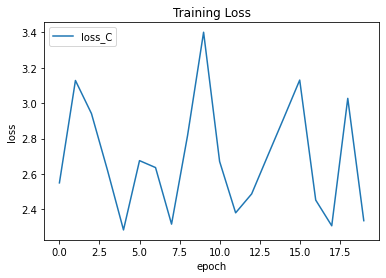# Training loss doesn't decreasing in CNN

Hello,

I trained a CNN model to categorizing 20 kinds of dogs in Stanford Dogs dataset.

However, I cannot reduce the loss value.

There are some parts of my codes. Can anyone help?

``````train_dataloader, test_dataloader = split_Train_Val_Data(data_dir)

C = models.resnet50(num_classes=20).to(device)
optimizer_C = optim.Adam(C.parameters(), lr = 1e-4)

criteron = nn.CrossEntropyLoss()

``````
``````if __name__ == '__main__':

for epoch in range(epochs):

iter = 0
correct_train, total_train = 0, 0
correct_test, total_test = 0, 0
train_loss_C = 0.0

print('epoch: ' + str(epoch + 1) + ' / ' + str(epochs))

C.train()
for i, (x, label) in enumerate(train_dataloader) :
x, label = x.to(device), label.to(device)

output = C(x)
loss = criteron(output, label.long())

loss = Variable(loss, requires_grad = True)
loss.backward()
optimizer_C.step()

_, predicted = torch.max(output.data, 1)
total_train += len(x)
correct_train += (predicted==label).sum().item()

# train_loss_C += loss.item()*len(label)
train_loss_C += loss.item()
iter += 1

print('Training epoch: %d / loss_C: %.3f | acc: %.3f' % \
(epoch + 1, train_loss_C / iter, correct_train / total_train))

C.eval()
for i, (x, label) in enumerate(test_dataloader) :

x, label = x.to(device), label.to(device)

output = C(x)
loss = criteron(output, label.long())
_, predicted = torch.max(output.data, 1)
total_test += len(x)
correct_test += (predicted==label).sum().item()

print('Testing acc: %.3f' % (correct_test / total_test))

train_acc.append(100 * correct_train/total_train)
test_acc.append(100 * correct_test/total_test)
loss_epoch_C.append(train_loss_C)
``````You are disabling the gradient calculation and are rewrapping the `loss` tensor thus detaching it from the graph in these lines of code:

``````           with torch.no_grad():
output = C(x)
loss = criteron(output, label.long())

loss = Variable(loss, requires_grad = True)
``````

Remove the `with torch.no_grad()` guard and don’t recreate the `loss` tensor.

Also, `Variable`s are deprecated since PyTorch `0.4` so you can use tensors now.

1 Like